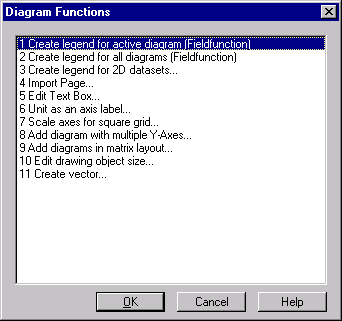# Diagram=>More Diagram Functions¶Scale Axes for Square Grid
Scales the axes for all diagrams on the active page so that a net with defined facets width is created (see Diagram=>More Diagram Functions=>Scale Axes for Square Grid).
Inserts a diagram with multiple y-axes to the active page (see Stacked diagrams with multiple y axes).
Inserts multiple diagrams in matrix layout to the active page (see Diagram=>Add Diagrams (Matrix)).
Create Legend for 2D Datasets
Creates a legend for the selected diagram or for all diagrams (see Diagram=>More Diagram Functions=>Create Legend for 2D Datasets).
Unit as Axis Label
Replaces the second last label with text (see Diagram=>More Diagram Functions=>Unit as Axis Label).
Import Page
Adds the elements of a UniPlot document page to the active page (see Diagram=>More Diagram Functions=>Import Page).
Edit Text Box
How you can create your own text boxes and add them to the active page see Diagram=>More Diagram Functions=>Import Page
Edit Drawing Object Size
Edits the size of the selected drawing object (see Diagram=>More Diagram Functions=>Edit Drawing Object Size).
Create Vector
Creates a dataset with 2 points and connects the points with a straight line (see Diagram=>More Diagram Functions=>Create Vector).